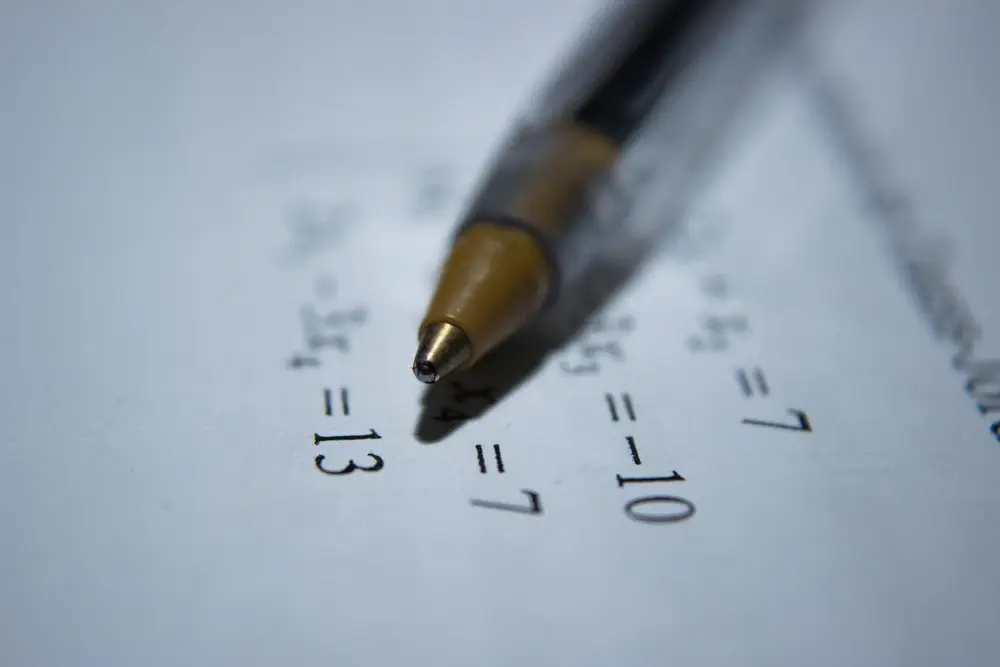# Indices

An index is the number which is at the top right hand corner of a figure which denotes a power. The power of a number is how many times the number is multiplied by itself. For example:

22 = 2 × 2 = 4
43 = 4 × 4 × 4 = 64
34 = 3 × 3 × 3 × 3 = 81

When using indices you should remember the following things:

• nx × ny = nx+y

Division subtracts the powers:

• nx ÷ ny = nx-y

A negative index means the number is reciprocated:
n-x = 1/nx

For fractional indices, the numerator is the power of the base number and the denominator is the root of this number:
nx/y = y

nx

Also, remember the following:
n1 = n
n0 = 1

Algebra

Algebra

Algebra

Algebra

Algebra

Algebra